# •Bible Code Software•

Matrices >> Sports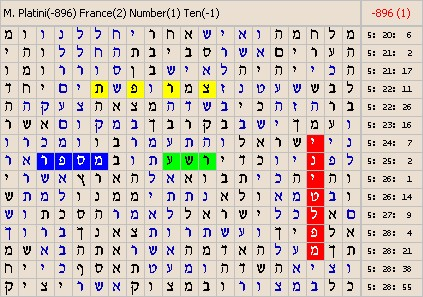The skip of the matrix is -896.
The masterword of the matrix is : M. Platini.
The slavewords of the matrix are : France, Number, Ten.
Wikipedia : PlatiniThe skip of the matrix is 21.
The masterword of the matrix is : U. Bolt.
The slavewords of the matrix are : 9.69, the record holder, 19.30, time.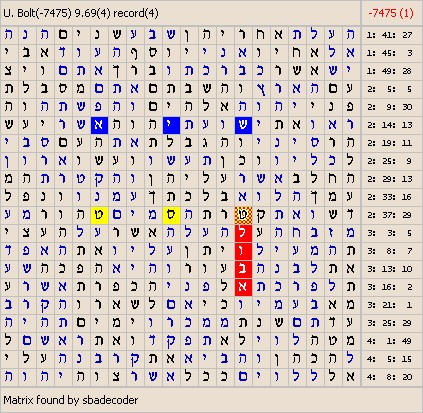The skip of the matrix is -7475.
The masterword of the matrix is : U. Bolt.
The slavewords of the matrix are : 9.69, record.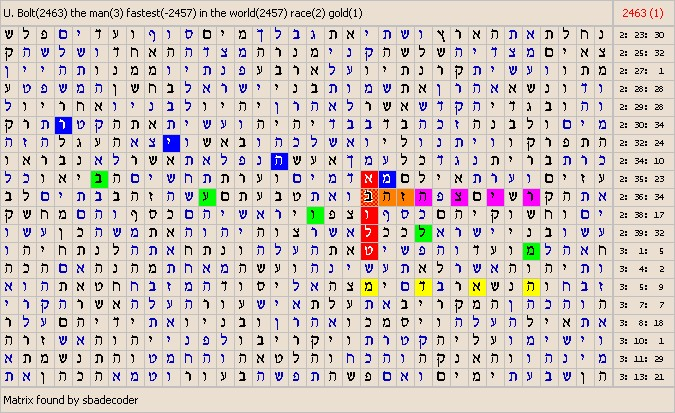The skip of the matrix is 2463.
The masterword of the matrix is : U. Bolt.
The slavewords of the matrix are : the man, fastest, in the world, race, gold.
Wikipedia : BoltThe skip of the matrix is 32.
The masterword of the matrix is : Schumacher.
The slavewords of the matrix are : accident, ski, ski.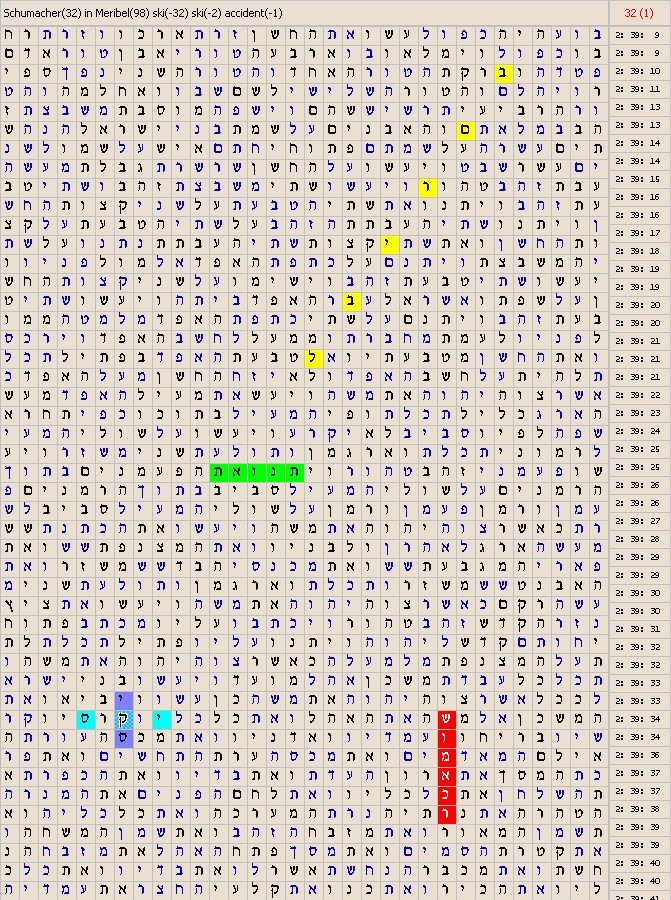The skip of the matrix is 32.
The masterword of the matrix is : Schumacher.
The slavewords of the matrix are : accident, ski, ski, in Meribel.
Wikipedia : Schumacher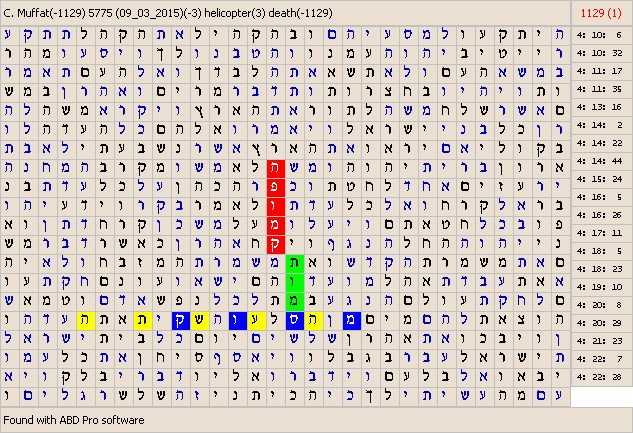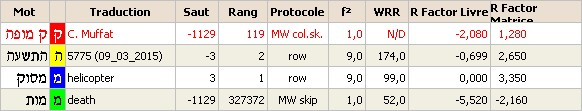The skip of the matrix is -1129.
The masterword of the matrix is : C. Muffat.
The slavewords of the matrix are : helicopter, 5775(09_03_2015), death.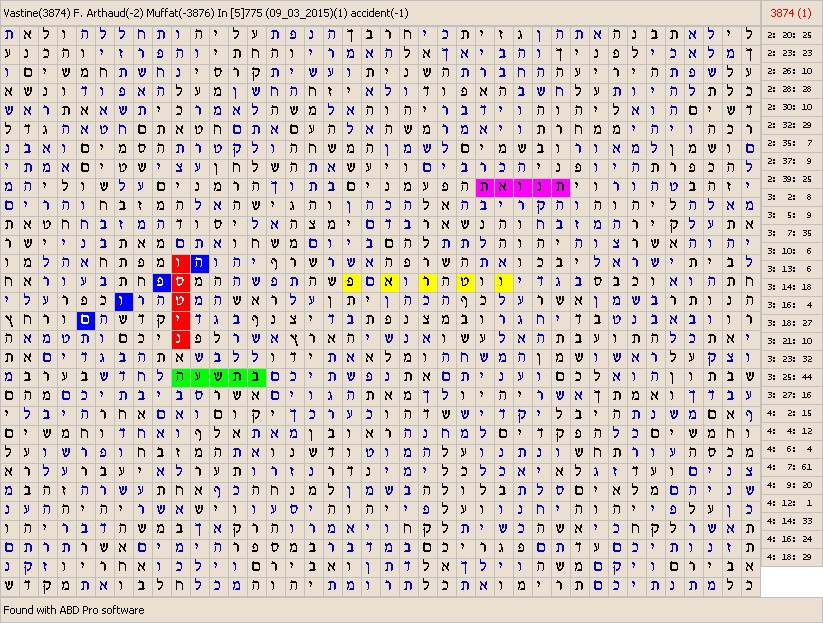The skip of the matrix is 3874.
The masterword of the matrix is : Vastine.
The slavewords of the matrix are : F. Arthaud, Muffat, accident, in 775 (09_03_2015).
Wikipedia : Alexis Vastine Wikipedia : Camille Muffat Wikipedia : Florence Arthaud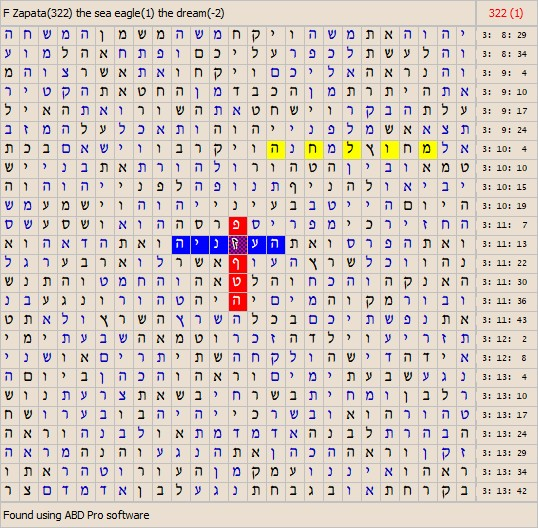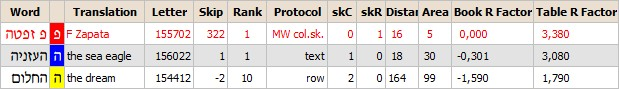The skip of the matrix is 322.
The masterword of the matrix is : F. Zapata.
The slavewords of the matrix are : the sea eagle, the dream.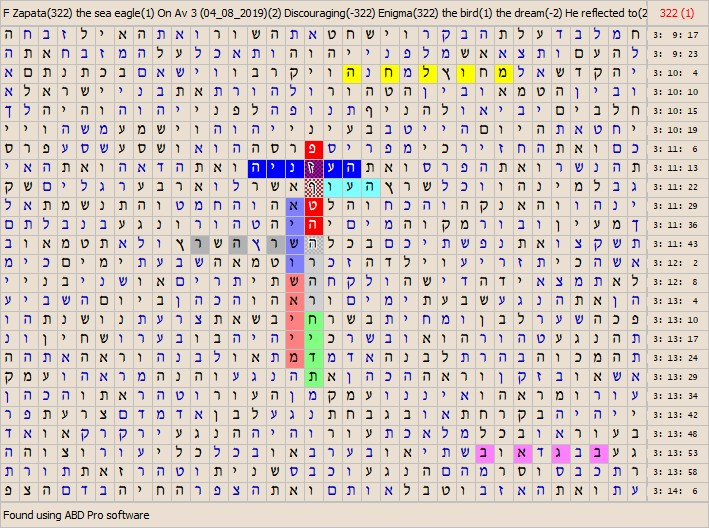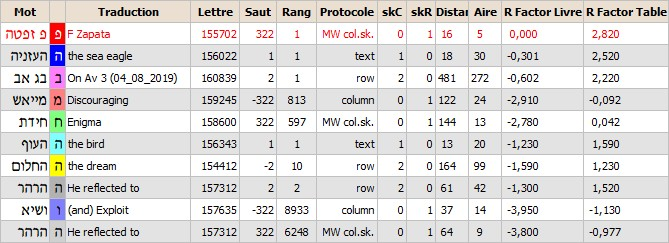The skip of the matrix is 322.
The masterword of the matrix is : F. Zapata.
The slavewords of the matrix are : the sea eagle, the bird, he reflected to, Enigma, the dream, Discouraging, (and) Exploit, On Av 3 (04-08-2019), .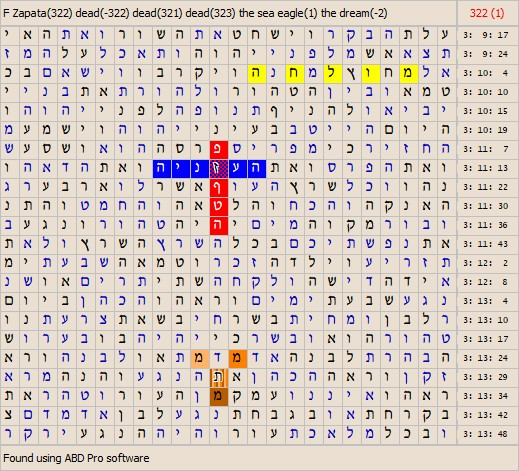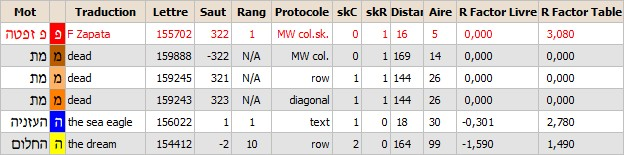The skip of the matrix is 322.
The masterword of the matrix is : F. Zapata.
The slavewords of the matrix are : the sea eagle, the dream, dead?.
Wikipedia : Franky Zapata